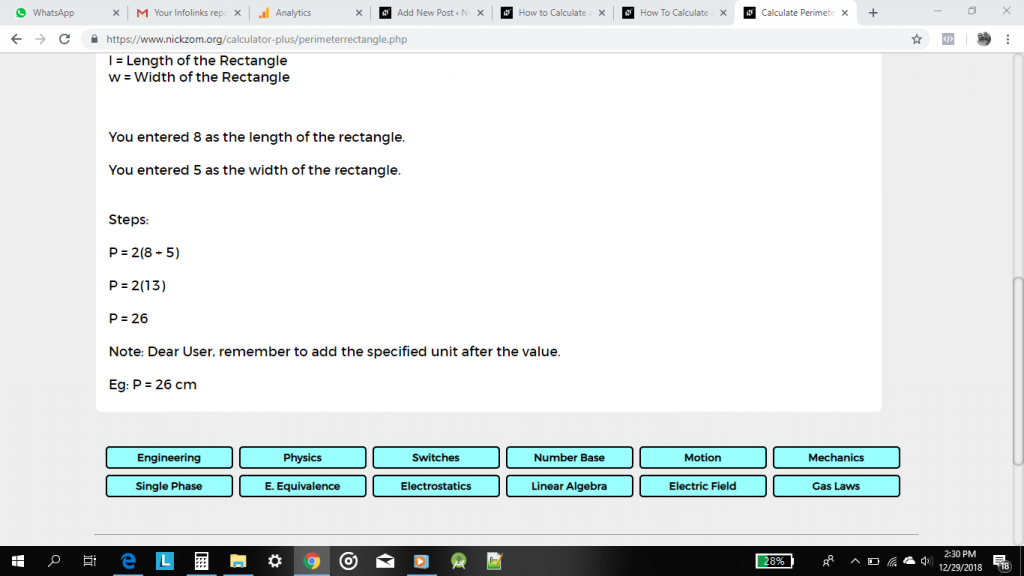# How to Calculate and Solve for the Perimeter, Length and Width of a Rectangle | The Calculator EncyclopediaThe image above is a rectangle with a length of 8 cm and a width of 5 cm.
To compute the perimeter of a rectangle requires two parameters which are the length of the rectangle and the width of the rectangle.

The formula for calculating the perimeter of a rectangle is:

P = 2 (l + w)

Where:

P = Perimeter of a Rectangle
l = Length of a Rectangle
w = Width of a Rectangle

Let’s solve an example:
Find the perimeter of a rectangle where the length of the rectangle is 8 cm and the width of the rectangle is 5 cm.

This implies that:
l = Length of the Rectangle = 8 cm
w = Width of the Rectangle = 5 cm

P = 2 (l + w)
P = 2 (8 + 5)
P = 2 (13)
P = 26

Therefore, the perimeter of the rectangle is 26 cm.

How to Calculate the Length of a Rectangle when the Perimeter of the Rectangle and the Width of the Rectangle is Given

l = P / 2 – w

Where:
l = Length of the Rectangle
P = Perimeter of the Rectangle
w = Width of the Rectangle

Let’s solve an example:
Find the length of a rectangle where the perimeter of the rectangle is 36 cm and the width of the rectangle is 6 cm.

This implies that:
P = Perimeter of the Rectangle = 36 cm
w = Width of the Rectangle = 6 cm

l = P / 2 – w
l = 36 / 2 – 6
l = 18 – 6
l = 12

Therefore, the length of the rectangle is 12 cm.

How to Calculate the Width of a Rectangle when the Perimeter of the Rectangle and the Length of the Rectangle is Given

w = P / 2 – l

Where:
w = Width of the Rectangle
P = Perimeter of the Rectangle
l = Length of the Rectangle

Let’s solve an example:
Find the width of a rectangle where the perimeter of the rectangle is 120 cm and the length of the rectangle is 10 cm.

This implies that:
P = Perimeter of the Rectangle = 120 cm
l = Length of the Rectangle = 10 cm

w = P / 2 – l
w = 120 / 2 – 10
w = 60 – 10
w = 50

Therefore, the width of the rectangle is 50 cm.

Nickzom Calculator – The Calculator Encyclopedia is capable of calculating the perimeter of a rectangle.

To get the answer and workings of the perimeter of a rectangle using the Nickzom Calculator – The Calculator Encyclopedia. First, you need to obtain the app.

You can get this app via any of these means:

To get access to the professional version via web, you need to register and subscribe for NGN 1,500 per annum to have utter access to all functionalities.
You can also try the demo version via https://www.nickzom.org/calculator

Once, you have obtained the calculator encyclopedia app, proceed to the Calculator Map, then click on Mensuration under the Mathematics sectionNow, click on Perimeter of a Rectangle under MensurationThe screenshot below displays the page or activity to enter your values, to get the answer for the perimeter of a rectangle according to the respective parameters which are the length of the rectangle (l) and the width of the rectangle (w).Now, enter the values appropriately and accordingly for the parameters as required by the example above where the length of the rectangle is 8 cm and the width of the rectangle is 5 cm.Finally, click on the Calculate buttonAs you can see from the screenshot above, Nickzom Calculator – The Calculator Encyclopedia solves for the perimeter of a rectangle and presents the formula, workings and steps too.